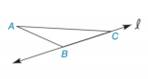Chapter 2.6, Problem 13E### Elementary Geometry for College St...

6th Edition
Daniel C. Alexander + 1 other
ISBN: 9781285195698

#### Solutions

Chapter
Section### Elementary Geometry for College St...

6th Edition
Daniel C. Alexander + 1 other
ISBN: 9781285195698
Textbook Problem
1 views

# Complete each figure so that it reflects across line l .To determine

a)

To draw:

The complete figure symmetrical to line 𝓁.

Explanation

Definition Used:

The figure to be reflected across the given line this implies that for every point A on the left side of the line, there should be second point A such that line should act as the perpendicular bisector of AA¯

To determine

b)

To draw:

The complete figure symmetrical to line 𝓁.

### Still sussing out bartleby?

Check out a sample textbook solution.

See a sample solution

#### The Solution to Your Study Problems

Bartleby provides explanations to thousands of textbook problems written by our experts, many with advanced degrees!

Get Started

#### Find the distance between the points. 4. (1, 6), (1, 3)

Single Variable Calculus: Early Transcendentals, Volume I

#### In Exercises 1-6, simplify the expression. 27+12

Calculus: An Applied Approach (MindTap Course List)

#### Use sets toshow that 06=0.

Mathematical Excursions (MindTap Course List)

#### EXPLORING CONCEPTS f(x)=2xx2+1,[0,4] (a) 3 (b) 7 (c) -2 (d) 5 (e) 1

Calculus: Early Transcendental Functions (MindTap Course List)